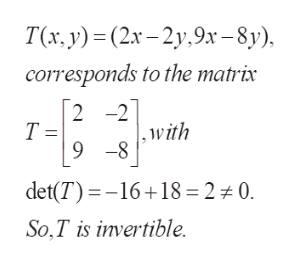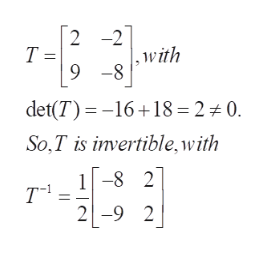Linear Alegebra: Show that the transformation t(x1,x2)=(2x1-2x2,9x1-8x2) is invertible, and find a formula for T^-1.

Question

Linear Alegebra: Show that the transformation t(x1,x2)=(2x1-2x2,9x1-8x2) is invertible, and find a formula for T^-1.

Step 1

To prove that the given linear transformation is invertible and to find the inverze

Step 2

To avoid suffixes, use x and y . The matrix of T has determinant not zero. So it is invertiblehelp_outlineImage TranscriptioncloseT(x., у) - (2х - 2у.9х - 8). corresponds to the matrix 2 -2 Т- 9 -8 ,with det(T)16+18 = 2 0. So,T is invertible. fullscreen
Step 3

T inverse is found by the standard formula fo...help_outlineImage Transcriptionclose2 -2 Т— 9 -8 , with det(T)16 18 = 2 0. So,T is invertible,with 1-8 2 Tч 2-9 fullscreen

Want to see the full answer?

See Solution

Want to see this answer and more?

Our solutions are written by experts, many with advanced degrees, and available 24/7

See Solution
Tagged in

Math